JMSLTM Numerical Library 7.2.0
com.imsl.math

## Class FFT

• All Implemented Interfaces:
Serializable, Cloneable

```public class FFT
extends Object
implements Serializable, Cloneable```
FFT functions.

Class `FFT` computes the discrete Fourier transform of a real vector of size n. The method used is a variant of the Cooley-Tukey algorithm, which is most efficient when n is a product of small prime factors. If n satisfies this condition, then the computational effort is proportional to n log n.

The `forward` method computes the forward transform. If n is even, then the forward transform is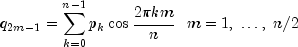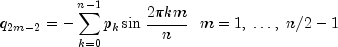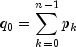If n is odd,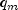is defined as above for m from 1 to (n - 1)/2.

Let f be a real valued function of time. Suppose we sample f at n equally spaced time intervals of length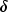seconds starting at time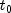. That is, we have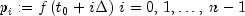We will assume that n is odd for the remainder of this discussion. The class `FFT` treats this sequence as if it were periodic of period n. In particular, it assumes that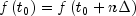. Hence, the period of the function is assumed to be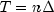. We can invert the above transform for p as follows: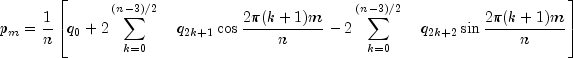This formula is very revealing. It can be interpreted in the following manner. The coefficients q produced by `FFT` determine an interpolating trigonometric polynomial to the data. That is, if we define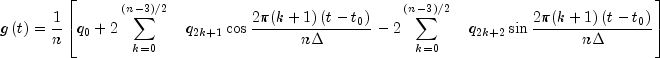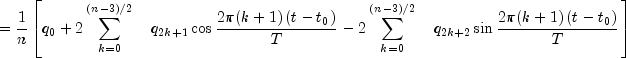then we have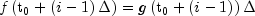Now suppose we want to discover the dominant frequencies, forming the vector P of length (n + 1)/2 as follows: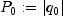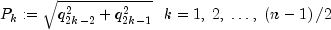These numbers correspond to the energy in the spectrum of the signal. In particular,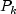corresponds to the energy level at frequency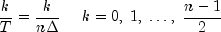Furthermore, note that there are only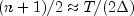resolvable frequencies when n observations are taken. This is related to the Nyquist phenomenon, which is induced by discrete sampling of a continuous signal. Similar relations hold for the case when n is even.

If the `backward` method is used, then the backward transform is computed. If `n` is even, then the backward transform is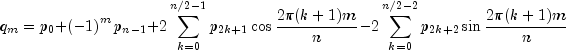If n is odd,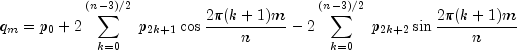The backward Fourier transform is the unnormalized inverse of the forward Fourier transform.

`FFT` is based on the real FFT in FFTPACK, which was developed by Paul Swarztrauber at the National Center for Atmospheric Research.

Example, Serialized Form
• ### Constructor Summary

Constructors
Constructor and Description
`FFT(int n)`
Constructs an FFT object.
• ### Method Summary

Methods
Modifier and Type Method and Description
`double[]` `backward(double[] coef)`
Compute the real periodic sequence from its Fourier coefficients.
`double[]` `forward(double[] seq)`
Compute the Fourier coefficients of a real periodic sequence.
• ### Methods inherited from class java.lang.Object

`clone, equals, finalize, getClass, hashCode, notify, notifyAll, toString, wait, wait, wait`
• ### Constructor Detail

• #### FFT

`public FFT(int n)`
Constructs an FFT object.
Parameters:
`n` - is the length of the sequence to be transformed
• ### Method Detail

• #### backward

`public double[] backward(double[] coef)`
Compute the real periodic sequence from its Fourier coefficients.
Parameters:
`coef` - a `double` array containing the Fourier coefficients
Returns:
a `double` array containing the periodic sequence
• #### forward

`public double[] forward(double[] seq)`
Compute the Fourier coefficients of a real periodic sequence.
Parameters:
`seq` - a `double` array containing the sequence to be transformed
Returns:
a `double` array containing the transformed sequence
JMSLTM Numerical Library 7.2.0